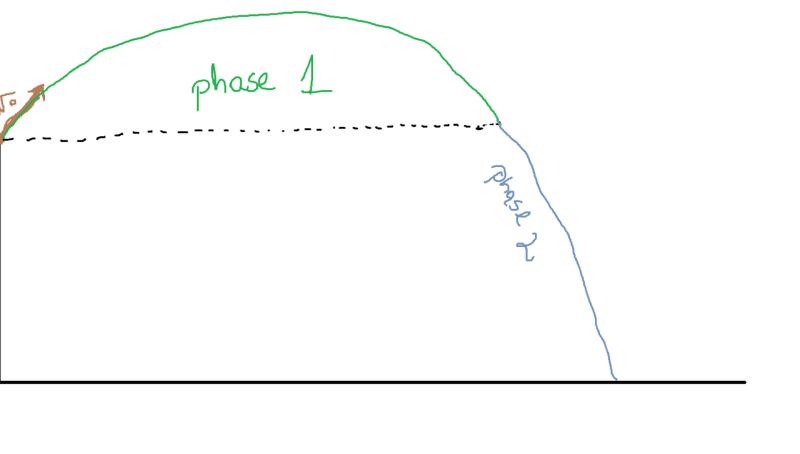# Shooting a bullet at an angle from a given height

## Homework Statement

A sniper standing on a 70 meter-tall tower fires his rifle at 30 degrees upwards with the initial velocity of 1000 m/s. What is the range of the bullet and how much time will pass before it hits the ground? Assume no air-resistance.

2. The attempt at a solution
I divided the motion of the bullet into two phases, the first one - until the bullets reaches the level at which it was released and the second one - from the end of phase 1, until it hits the ground. Therefore, in order to find the total distance the object will cover, I need to find the time each of these phases will take:
$$x_{max} = v_x(t_1+t_2)$$Phase 1:
Velocity:
$$\begin{cases} v_x = v_o \cos\theta \\ v_y = v_o \sin\theta - gt \end{cases}$$
Time:
$$t_1 = \frac{2v_o \sin\theta}{g}$$

Phase 2:
Velocity:
$$\begin{cases} v_x = v_o \cos\theta \\ v_y = -v_o \sin\theta - gt \end{cases}$$
Time:
In order to find the time the second phase will take, I calculate how much time it will take the object to fall from y = H to y = 0:
$$v_o \sin(\theta) t + \frac{1}{2}gt^2 = H \\ gt^2 + 2v_o \sin(\theta) t - 2H = 0 \\ t_2 = \frac{ \sqrt{v_o^2 \sin^2 \theta + 2gH} - v_o \sin \theta }{g}$$
And therefore the total time of the motion:
$$t = t_1 + t_2 = \frac{ \sqrt{v_o^2 \sin^2 \theta + 2gH} + v_o \sin \theta }{g}$$

Could you tell me if I've tackled this problem correctly?